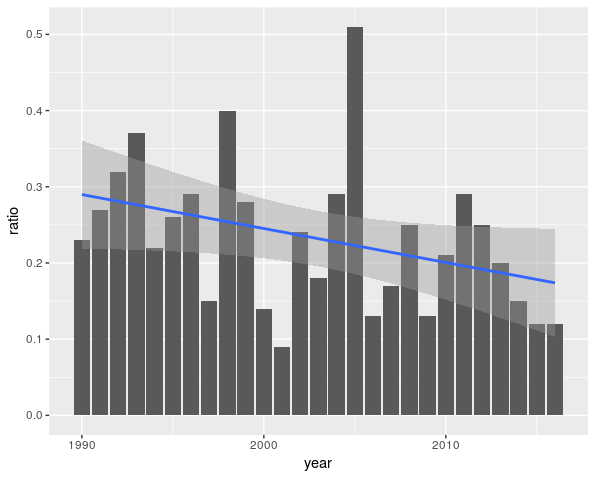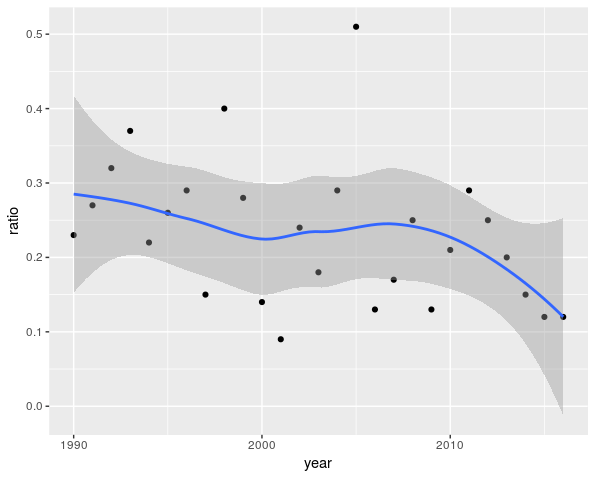Want to share your content on R-bloggers? click here if you have a blog, or here if you don't.

I had a very stranger discussion on twitter (yes, another one), about regression curves. I think it started with a tweet based on some xkcd picture (just for fun, because it was New Year’s Day)

There were comments on that picture, by econometricians, mainly about ‘significant’ trends when datasets are very noisy. And I mentioned a graph that I saw earlier, that day

Let us reproduce that graph (Roger kindly sent me the dataset)

db=data.frame(year=1990:2016, ratio=c(.23,.27,.32,.37,.22,.26,.29,.15,.40,.28,.14,.09,.24,.18,.29,.51,.13,.17,.25,.13,.21,.29,.25,.2,.15,.12,.12)) library(ggplot2)

The graph is here (using the same conventions as Roger’s initial graph, using some sort of barplot)

ggplot(db, aes(year, ratio)) + geom_bar(stat="identity") + stat_smooth(method = "lm", se = FALSE)My point was that we miss the ‘confidence band’ of the regression

In R, at least, it is quite natural to get (and actually, it is the default version of the graph function)

ggplot(db, aes(year, ratio)) + geom_bar(stat="identity") + stat_smooth(method = "lm", se = TRUE)It is hard to claim that the ‘regression line’ is significant (in the sense significantly non horizontal). To be more specific, if we look at the output of the regression model, we get

summary(lm(ratio~year,data=db)) 

Coefficients: Estimate    Std. Error t value Pr(>|t|) (Intercept) 9.158531 4.549672  2.013 0.055 . year       -0.004457 0.002271 -1.962 0.061 . --- Signif. codes: 0.01 ‘*’ 0.05 ‘.’ 0.1 ‘ ’ 1

(which is exactly what Roger used in his graph to plot his red straight line). The p-value of the estimator of the slope, in a linear regression model is here 6%. But I found Roger’s point puzzeling

First of all, let us get back to a more standard graph, with a scatterplot, and not bars,

ggplot(db, aes(year, ratio)) + stat_smooth(method = "lm") + geom_point()Here, we observe points . In order to draw that blue line, we assume (econometrics 101) that those observations are realizations of random variables . Randomness here does not come from a survey, or from ‘balls in an urn’. Randomness is because hurricanes and floods are themselves seen are realizations of random events. Yes, there might be measurement errors, but that’s not where randomness comes from. Here, when we talk about ‘randomness’, it should be related to ‘model error’ i.e. the error we make if we consider a linear model (here), that is

Even if observations are not obtained from balls in an urn, there is some kind of randomness here. One might consider a nonlinear model to reduce the error,

ggplot(db, aes(year, ratio)) + geom_point() + geom_smooth()

but in the case, the danger is to overfit